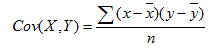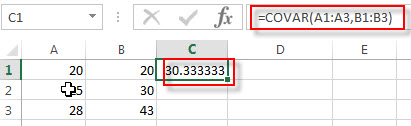# Excel COVAR Function

This post will guide you how to use the COVAR function with syntax and examples in Microsoft excel.

### Description

The Excel COVAR function returns covariance, the average of the products of deviations for each data point in two given sets of values. So you can use the COVAR function to calculate the covariance of two data sets in Excel .

The covariance is a statistical measurement of the strength of the correlation between two data sets.

The COVAR function is a build-in function in Microsoft Excel and it is categorized as a Statistical Function.

The COVAR function is available in Excel 2016, Excel 2013, Excel 2010, Excel 2007, Excel 2003, Excel XP, Excel 2000, Excel 2011 for Mac. And this function has been replaced by the COVARIANCE.P function in Excel 2010 version. But it is still available in Excel 2010 or earlier version of Excel.

### Syntax

The syntax of the COVAR function is as below:

`= COVAR (array1,array2)`

Where the COVAR function arguments are:

• array1 – This is a required argument.  The first cell range of integers. The array must contain numeric values.
• array2 – This is a required argument.  The second cell range of integers. The array must contain numeric values.

Note:

• The arguments must be numbers or arrays or references that contain numeric values.
• If any arguments contain text, logical values, or empty cells, and those values will be ignored by the COVAR function.
• If Array1 and Array2 have different numbers of data sets, the COVAR function will return #N/A Error. Or if the two given arrays have different lengths, returns #N/A Error value.
• If either array1 or array2 argument is empty, the COVAR function will return the #DIV/0! Error value.
• the covariance is like the following equation:### Excel COVAR Function Examples

The below examples will show you how to use Excel COVAR Function to calculate the covariance of two given data sets.

1# to calculate the covariance, the average of the products of deviations for two supplied sets of values, using the following formula:

`=COVAR (A1:A3,B1:B3)`